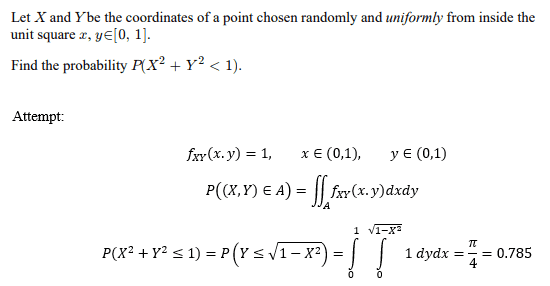# Probability/Random variables question

• ashah99

#### ashah99

Homework Statement
Finding the probability of randomly choosing a point within the unit square constrained within the quarter circle
Relevant Equations
P(( X, Y ) ∈ A) = ∫∫ fXY ( x, y )dxdy
Hello all, I am wondering if my approach is coreect for the following probability question? I believe the joint PDF would be 1 given that the point is chosen from the unit square. To me, this question can be reduced down to finding the area of 1/4 of a circle with radius 1. Any help is appreciated!It's clear from your calculations that you are indeed simply calculating the area. Which is what you would expect for a uniform distribution.

It's clear from your calculations that you are indeed simply calculating the area. Which is what you would expect for a uniform distribution.
Ok, makes sense. Would you agree that my answer is correct? Just want to make sure I understand.

Ok, makes sense. Would you agree that my answer is correct? Just want to make sure I understand.
Yes, it's just a ratio of areas, as you've calculated.

•ashah99
Or use polar coordinates: $$\int_0^1 \int_0^{\sqrt{1-x^2}} 1\,dy\,dx = \int_0^{\pi/2}\int_0^1 r\,dr\,d\theta.$$

•ashah99